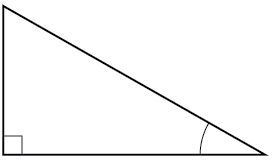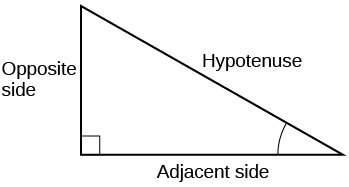# 5.4 Right triangle trigonometry  (Page 5/12)

 Page 5 / 12

## Measuring a distance indirectly

To find the height of a tree, a person walks to a point 30 feet from the base of the tree. She measures an angle of $57°\text{\hspace{0.17em}}$ between a line of sight to the top of the tree and the ground, as shown in [link] . Find the height of the tree.

We know that the angle of elevation is $\text{\hspace{0.17em}}57°\text{\hspace{0.17em}}$ and the adjacent side is 30 ft long. The opposite side is the unknown height.

The trigonometric function relating the side opposite to an angle and the side adjacent to the angle is the tangent. So we will state our information in terms of the tangent of $57°,$ letting $\text{\hspace{0.17em}}h\text{\hspace{0.17em}}$ be the unknown height.

The tree is approximately 46 feet tall.

How long a ladder is needed to reach a windowsill 50 feet above the ground if the ladder rests against the building making an angle of $\text{\hspace{0.17em}}\frac{5\pi }{12}\text{\hspace{0.17em}}$ with the ground? Round to the nearest foot.

About 52 ft

Access these online resources for additional instruction and practice with right triangle trigonometry.

Visit this website for additional practice questions from Learningpod.

## Key equations

 Cofunction Identities $\begin{array}{l}\begin{array}{l}\\ \mathrm{cos}\text{\hspace{0.17em}}t=\mathrm{sin}\left(\frac{\pi }{2}-t\right)\end{array}\hfill \\ \mathrm{sin}\text{\hspace{0.17em}}t=\mathrm{cos}\left(\frac{\pi }{2}-t\right)\hfill \\ \mathrm{tan}\text{\hspace{0.17em}}t=\mathrm{cot}\left(\frac{\pi }{2}-t\right)\hfill \\ \mathrm{cot}\text{\hspace{0.17em}}t=\mathrm{tan}\left(\frac{\pi }{2}-t\right)\hfill \\ \mathrm{sec}\text{\hspace{0.17em}}t=\mathrm{csc}\left(\frac{\pi }{2}-t\right)\hfill \\ \mathrm{csc}\text{\hspace{0.17em}}t=\mathrm{sec}\left(\frac{\pi }{2}-t\right)\hfill \end{array}$

## Key concepts

• We can define trigonometric functions as ratios of the side lengths of a right triangle. See [link] .
• The same side lengths can be used to evaluate the trigonometric functions of either acute angle in a right triangle. See [link] .
• We can evaluate the trigonometric functions of special angles, knowing the side lengths of the triangles in which they occur. See [link] .
• Any two complementary angles could be the two acute angles of a right triangle.
• If two angles are complementary, the cofunction identities state that the sine of one equals the cosine of the other and vice versa. See [link] .
• We can use trigonometric functions of an angle to find unknown side lengths.
• Select the trigonometric function representing the ratio of the unknown side to the known side. See [link] .
• Right-triangle trigonometry permits the measurement of inaccessible heights and distances.
• The unknown height or distance can be found by creating a right triangle in which the unknown height or distance is one of the sides, and another side and angle are known. See [link] .

## Verbal

For the given right triangle, label the adjacent side, opposite side, and hypotenuse for the indicated angle.When a right triangle with a hypotenuse of 1 is placed in the unit circle, which sides of the triangle correspond to the x - and y -coordinates?

The tangent of an angle compares which sides of the right triangle?

The tangent of an angle is the ratio of the opposite side to the adjacent side.

What is the relationship between the two acute angles in a right triangle?

Explain the cofunction identity.

For example, the sine of an angle is equal to the cosine of its complement; the cosine of an angle is equal to the sine of its complement.

#### Questions & Answers

I've run into this: x = r*cos(angle1 + angle2) Which expands to: x = r(cos(angle1)*cos(angle2) - sin(angle1)*sin(angle2)) The r value confuses me here, because distributing it makes: (r*cos(angle2))(cos(angle1) - (r*sin(angle2))(sin(angle1)) How does this make sense? Why does the r distribute once
Carlos Reply
How can you tell what type of parent function a graph is ?
Mary Reply
generally by how the graph looks and understanding what the base parent functions look like and perform on a graph
William
if you have a graphed line, you can have an idea by how the directions of the line turns, i.e. negative, positive, zero
William
y=x will obviously be a straight line with a zero slope
William
y=x^2 will have a parabolic line opening to positive infinity on both sides of the y axis vice versa with y=-x^2 you'll have both ends of the parabolic line pointing downward heading to negative infinity on both sides of the y axis
William
y=x will be a straight line, but it will have a slope of one. Remember, if y=1 then x=1, so for every unit you rise you move over positively one unit. To get a straight line with a slope of 0, set y=1 or any integer.
Aaron
yes, correction on my end, I meant slope of 1 instead of slope of 0
William
what is f(x)=
Karim Reply
I don't understand
Joe
Typically a function 'f' will take 'x' as input, and produce 'y' as output. As 'f(x)=y'. According to Google, "The range of a function is the complete set of all possible resulting values of the dependent variable (y, usually), after we have substituted the domain."
Thomas
Sorry, I don't know where the "Â"s came from. They shouldn't be there. Just ignore them. :-)
Thomas
GREAT ANSWER THOUGH!!!
Darius
Thanks.
Thomas
Â
Thomas
It is the Â that should not be there. It doesn't seem to show if encloses in quotation marks. "Â" or 'Â' ... Â
Thomas
Now it shows, go figure?
Thomas
what is this?
unknown Reply
i do not understand anything
unknown
lol...it gets better
Darius
I've been struggling so much through all of this. my final is in four weeks 😭
Tiffany
this book is an excellent resource! have you guys ever looked at the online tutoring? there's one that is called "That Tutor Guy" and he goes over a lot of the concepts
Darius
thank you I have heard of him. I should check him out.
Tiffany
is there any question in particular?
Joe
I have always struggled with math. I get lost really easy, if you have any advice for that, it would help tremendously.
Tiffany
Sure, are you in high school or college?
Darius
Hi, apologies for the delayed response. I'm in college.
Tiffany
how to solve polynomial using a calculator
Ef Reply
So a horizontal compression by factor of 1/2 is the same as a horizontal stretch by a factor of 2, right?
KARMEL Reply
The center is at (3,4) a focus is at (3,-1), and the lenght of the major axis is 26
Rima Reply
The center is at (3,4) a focus is at (3,-1) and the lenght of the major axis is 26 what will be the answer?
Rima
I done know
Joe
What kind of answer is that😑?
Rima
I had just woken up when i got this message
Joe
Can you please help me. Tomorrow is the deadline of my assignment then I don't know how to solve that
Rima
i have a question.
Abdul
how do you find the real and complex roots of a polynomial?
Abdul
@abdul with delta maybe which is b(square)-4ac=result then the 1st root -b-radical delta over 2a and the 2nd root -b+radical delta over 2a. I am not sure if this was your question but check it up
Nare
This is the actual question: Find all roots(real and complex) of the polynomial f(x)=6x^3 + x^2 - 4x + 1
Abdul
@Nare please let me know if you can solve it.
Abdul
I have a question
juweeriya
hello guys I'm new here? will you happy with me
mustapha
The average annual population increase of a pack of wolves is 25.
Brittany Reply
how do you find the period of a sine graph
Imani Reply
Period =2π if there is a coefficient (b), just divide the coefficient by 2π to get the new period
Am
if not then how would I find it from a graph
Imani
by looking at the graph, find the distance between two consecutive maximum points (the highest points of the wave). so if the top of one wave is at point A (1,2) and the next top of the wave is at point B (6,2), then the period is 5, the difference of the x-coordinates.
Am
you could also do it with two consecutive minimum points or x-intercepts
Am
I will try that thank u
Imani
Case of Equilateral Hyperbola
Jhon Reply
ok
Zander
ok
Shella
f(x)=4x+2, find f(3)
Benetta
f(3)=4(3)+2 f(3)=14
lamoussa
14
Vedant
pre calc teacher: "Plug in Plug in...smell's good" f(x)=14
Devante
8x=40
Chris
Explain why log a x is not defined for a < 0
Baptiste Reply
the sum of any two linear polynomial is what
Esther Reply
divide simplify each answer 3/2÷5/4
Momo Reply
divide simplify each answer 25/3÷5/12
Momo
how can are find the domain and range of a relations
austin Reply
the range is twice of the natural number which is the domain
Morolake
A cell phone company offers two plans for minutes. Plan A: $15 per month and$2 for every 300 texts. Plan B: $25 per month and$0.50 for every 100 texts. How many texts would you need to send per month for plan B to save you money?
Diddy Reply
6000
Robert
more than 6000
Robert
For Plan A to reach $27/month to surpass Plan B's$26.50 monthly payment, you'll need 3,000 texts which will cost an additional \$10.00. So, for the amount of texts you need to send would need to range between 1-100 texts for the 100th increment, times that by 3 for the additional amount of texts...
Gilbert
...for one text payment for 300 for Plan A. So, that means Plan A; in my opinion is for people with text messaging abilities that their fingers burn the monitor for the cell phone. While Plan B would be for loners that doesn't need their fingers to due the talking; but those texts mean more then...
Gilbert

### Read also:

#### Get the best Precalculus course in your pocket!

Source:  OpenStax, Precalculus. OpenStax CNX. Jan 19, 2016 Download for free at https://legacy.cnx.org/content/col11667/1.6
Google Play and the Google Play logo are trademarks of Google Inc.

Notification Switch

Would you like to follow the 'Precalculus' conversation and receive update notifications?ByByBy Nick SwainByBy Mistry BhaveshBy